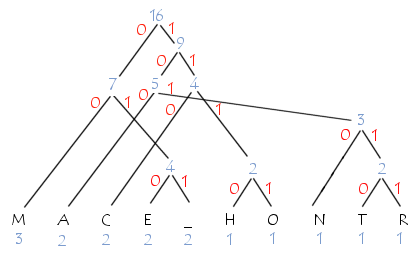# Question for a huffman tree

Hello everyone,
I would like to ask you a question, let’s imagine that we have a huffman tree below:we want to make a recursive scala function that wants to give us the number of bits to have a letter for example to have the letter ‘A’ with the tree of huffman it is necessary to have “1 0 0” for the letter ‘o’ it is necessary ‘1 1 1 1’ and the letter ‘h’ “1 1 1 0” and so on and we would like to make that in scala, we have already programmed a little but there are errors:

``````def encodeSymbol(c: Char, h: Huffman): Option[List[Bit]] = {
(h,c) match {
case (Feuille(x,y),c) => Some(Nil)
case (Noeud(x,y,z),c) =>
if(encodeSymbol(c,y).contains(c)){
One::encodeSymbol(c,y)}
else{Zero::encodeSymbol(c,z)}
}
}``````
``````sealed trait Huffman
case class Feuille(freq: Double, c: Char) extends Huffman
case class Noeud(freq: Double, zero: Huffman, one: Huffman) extends Huffman

sealed trait Bit
case object Zero extends Bit
case object One extends Bit``````

Could you be specific about your errors?

One error I see is that since `encodeSymbol(c, y)` is not a `List[Bit]` but an `Option[List[Bit]]`, you should have `encodeSymbol(c, y).map(One :: _ )` in place of `One :: encodeSymbol(c, y)`. The `else` case also needs the same correction.

Also `encodeSymbol(c,y).contains(c)` is probably not what you mean - it will check whether the option is non-empty and equals c (when you presumably mean the option is `Some(l)` and `l` contains `c`.

regards,
Siddhartha

To follow up on the logic of the `if` condition as I mentioned above, here is an example (from an ammonite REPL).

``````@ Some(List(1, 2)).contains(1)
res0: Boolean = false

@ Some(List(1, 2)).exists(_.contains(1))
res1: Boolean = true
``````

regards,
Siddhartha

Unrelated to your concrete question… For Huffman coding, once you have created the tree, you’d usually create a dictionary from chars to bit sequences once and then use this for encoding. (For decoding, you’d traverse the tree itself.)

I.e. you’d have something like

``````type HuffDict = Map[Char, List[Bit]]

def mkHuffDict(tree: Huffman): HuffDict = ???

def encodeSymbol(c: Char, dict: HuffDict): Option[List[Bit]] =
dict.get(c)
``````

Another thing: The frequencies are only required for creating the tree. Once you have the tree, they’re pretty much meaningless, so I’d consider excluding them from the tree nodes.

1 Like

First of all, thank you for your answer! The error I have is that I can’t return “One :: encodeSymbol(c,y)” and I don’t know how to do it

And it’s first time I see “.map”, what is its purpose and how does it work?

An option is a collection with at most one element, and `map` transforms each element of the collection. Here are a couple of examples with the collections of type `Option[Int]` and `List[Int]` which may make things clearer (otherwise perhaps you should look at a book or basic documentations). In the case of option, the unique element is transformed for the case where the option is non-empty, and an empty option gives an empty option.

``````@ val xOpt : Option[Int] = Some(2)
xOpt: Option[Int] = Some(2)

@ val yOpt : Option[Int] = None
yOpt: Option[Int] = None

@ xOpt.map(_ * 3)
res4: Option[Int] = Some(6)

@ yOpt.map(_ * 3)
res5: Option[Int] = None

@ List(1, 3, 5).map(_ * 3)
res6: List[Int] = List(3, 9, 15)
``````

regards,
Siddhartha

Oh right, it’s clearer now, thanks! But when I make the changes, it always returns “Some(List(Zero, Zero))” even though the character is not present in the tree, do you have a solution?

It would help if you post your whole code. As I see it, the above should be replaced by something like

``````if (encodeSymbol(c,z).exists(_.contains(c))){
encodeSymbol(c,z).map(One :: _)}
else if (encodeSymbol(c,y).exists(_.contains(c)))
{encodeSymbol(c,y).map(Zero :: _)}
else None
``````

There are also other ways to do this, perhaps more idiomatic.

regards,
Siddhartha

Hi all,

I don’t think this is appropriate to post the entire code.

This question is related to a graded programming exercise as part of an end-of-year project, and Wade, as a student, should rather ask his questions to his teachers, so that we can provide appropriate guidance without solving his problem entirely.

Thank you for your understanding.

1 Like